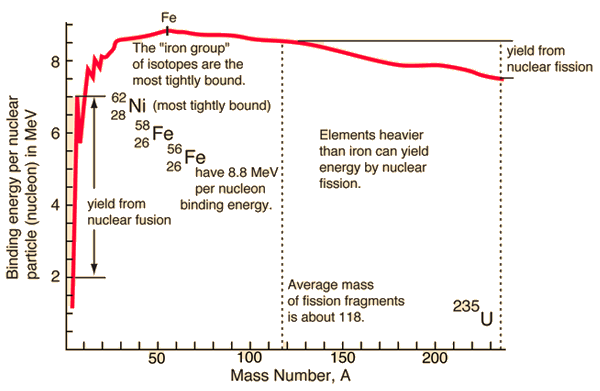-

### Activity & Half-Life

When a nucleus under goes decay it looses energy or mass. In the case of mass loss, changing from one isotope to another (and quiet often of a different element). It is impossible to predict which nucleus will decay in a sample. Every nucleus has the same probability of decaying, that is, the process of decay is completely random.

However, if there are enough nuclei in our sample then a fixed proportion will decay. The fixed proportion is given by $\lambda N$ where $\lambda$ is the probability of an individual nucleus decaying in a given time frame (usually per second) and $N$ is the number of original isotope nuclei and is known as the decay constant.

The decay rate can be measured and is called the activity, $A$ measured in Becquerel, Bq: $A=ΔNΔt$ where $t$ is the time taken, in seconds, to record the activity.

The activity is also given by $A=λ⁢N$ as the activity will be equal to the number of nuclei decaying.

Therefore, $-λ⁢N=ΔNΔt$ the negative sign indicating that this is a decay, i.e., the number of nuclei decreases.

Taking the limit as $t\to 0$ then this becomes $d N d t = - λ ⁢ N$

The solution to this equation is attained through integration: $N=N0⁢e-λ⁢t$ where $N$ is the number of the original nuclei remaining and ${N}_{0}$ is the initial number of nuclei of the particular isotope under consideration.

This is an exponential decay and when plotted gives a curve. Obtaining useful information about the decay from this curve can be more difficult than from a straight line, but a straight line form of the equation can be created by an algebraic process called linearisation. $N N0 = e-λ⁢t$ $ln N N0 = -λ⁢t$ $ln N - ln N0 = -λ⁢t$ $ln N = -λ⁢t + ln N0$ This is of the form $y=mx+c$ where $y=\mathrm{ln}N$, $m=-\lambda$, $x=t$ and $c=\mathrm{ln}{N}_{0}$. So plotting a graph of $\mathrm{ln}N$ versus $t$, the gradient give $-\lambda$ and the $y$-intercept gives ${N}_{0}$.

e.g. if there are 10000 nuclei present and 300 decay in 20 seconds, the decay constant is (300/10000)/20 = 0.0015s^-1.
Half-Life Equation
At time t=0, N=N0. At time T = t½ , N = 0.5N0. Substitute this into N=N0e(-λt):
Picture

This leads to the general form of the equation for half life:



A radioactive isotope may emit energy as photons of a specific energy, $E$. The power (energy transferred per second), $P=AE$, where $A$ is the number of decays per second. Half life, ${t}_{½}$ is the time taken for the number of the original isotopes to decrease to half the initial mass, or, time taken for the activity to half.

    the valley of nuclear stability